# Test: Basic Laws

## 10 Questions MCQ Test Topicwise Question Bank for Electronics Engineering | Test: Basic Laws

Description
Attempt Test: Basic Laws | 10 questions in 30 minutes | Mock test for Electrical Engineering (EE) preparation | Free important questions MCQ to study Topicwise Question Bank for Electronics Engineering for Electrical Engineering (EE) Exam | Download free PDF with solutions
QUESTION: 1

### The equivalent resistance of the circuit shown in figure below across the terminals a-b will be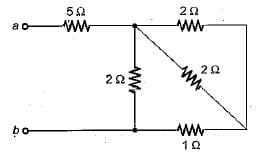Solution:

On combining the resitances in parallel, equivalent circuit will reduce as shown in below.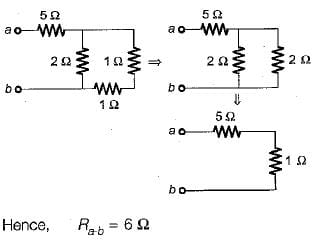QUESTION: 2

### For the circuit shown below, the equivalent resistance across terminals a-b will be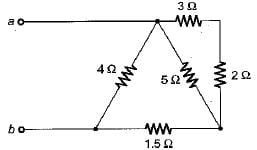Solution: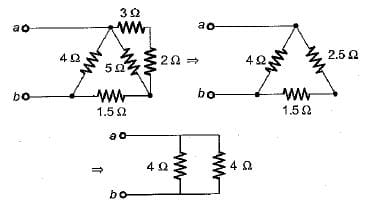Hence, equivalent resistance is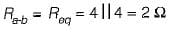QUESTION: 3

### What is the value of m so that the current I in the circuit shown below is maximum?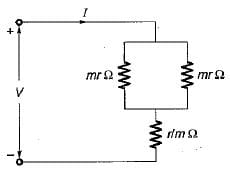Solution:

The equivalent resistance of the given circuit is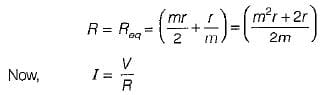Hence, for current I to be maximum , R should be minimum.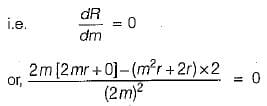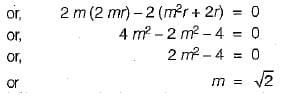QUESTION: 4

The given circuit shown below is converted to an equivalent voltage source V' connected in series with an equivalent resistance R'.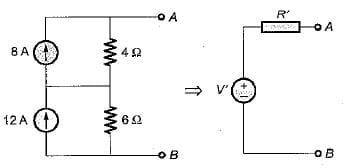The values of V' and R' are respectively

Solution:

Converting the current sources into equivalent voltage sources, the circuit is reduced as shown below.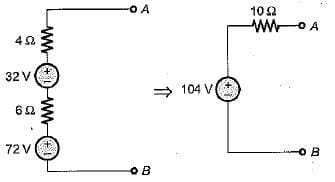QUESTION: 5

The equivalent current source for the circuit shown below will be represented as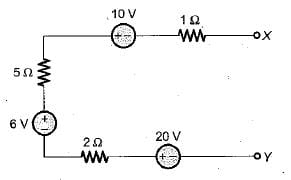Solution:

Equivalent voltage in the given circuit is

V= 6 + 2 0 - 1 0 = 16 volt

And equivalent resistance is R = 1 + 5 + 2 = 8 Ω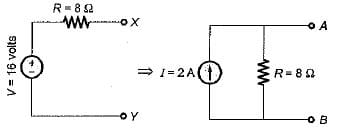QUESTION: 6

Assuming the batteries to be ideal voltage sources in the figure shown below, the value of current i is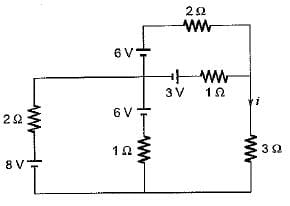Solution:

Converting all the voltage sources into equivalent current sources using source transformation, the equivalent circuit will be reudced as shown below.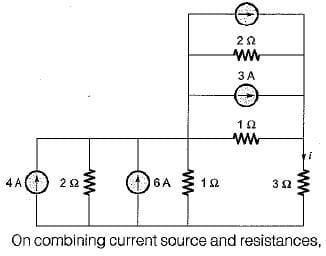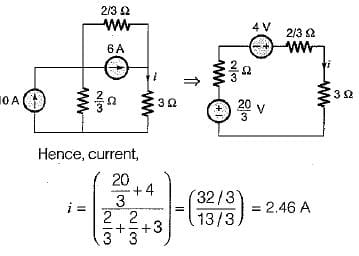QUESTION: 7

Two 100 W, 220 V bulbs are required to be connected across a 400 volts supply. The value of resistance to be inserted in the line so that the voltage across the bulbs does not exceed 220 V is

Solution:

Total power drawn from the circuit = 2 x 100 = 200 watts

Hence, supply current is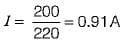Let R be the series resistance to be inserted in the circuit such that the voltage across the bulbs is 220 V.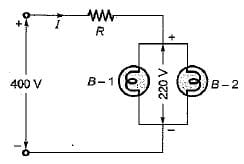Applying KVL , we get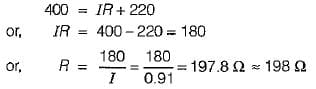QUESTION: 8

The equivalent resistance across the terminal X-Y for the circuit shown below is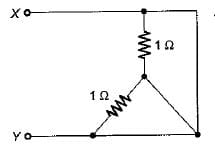Solution:

Converting the star-connected resistors into Δ equivalent, the given circuit is reduced as shown below.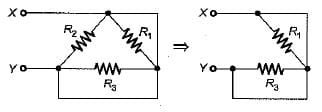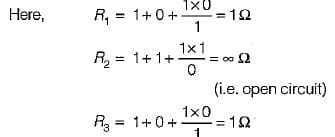Hence, equivalent resistance between terminals Xand Y is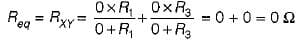QUESTION: 9

Assertion ( A ) : KCL states that at any node of a circuit, at any instant of time, the sum of incoming currents is equal to the sum of outgoing currents.
Reason (R): KCL indicates the law of conservation of energy.

Solution:

KCL is based on law of conservation of charge while KVL is based on law of conservation of energy.

QUESTION: 10

The total power absorbed in the circuit below is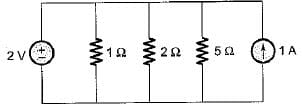Solution:

The current through the resistors will purely depend upon the voltage source of 2V connected a cross them . Let the currents i1 , i2  and i3 respectively flowing through the resistors of 1Ω,  2Ω and 5Ω.Use Code STAYHOME200 and get INR 200 additional OFF Use Coupon Code